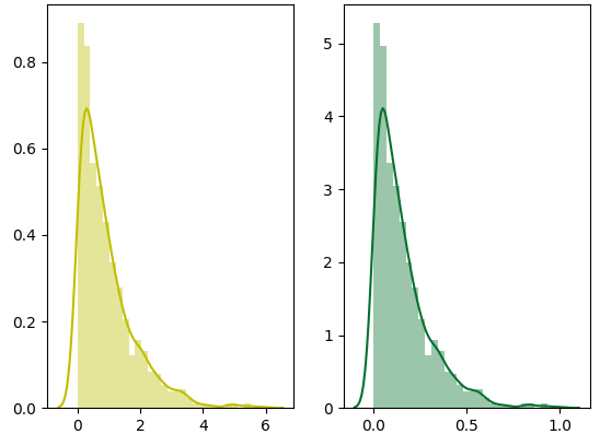RELATED TAGS

# Data normalization in PythonEducative Answers Team

Normalization refers to rescaling real-valued numeric attributes into a $0$ to $1$ range.

Data normalization is used in machine learning to make model training less sensitive to the scale of features. This allows our model to converge to better weights and, in turn, leads to a more accurate model.Left: Original Data, Right: Normalized Data

Normalization makes the features more consistent with each other, which allows the model to predict outputs more accurately.

## Code

Python provides the preprocessing library, which contains the normalize function to normalize the data. It takes an array in as an input and normalizes its values between $0$ and $1$. It then returns an output array with the same dimensions as the input.

from sklearn import preprocessing
import numpy as np

a = np.random.random((1, 4))
a = a*20
print("Data = ", a)

# normalize the data attributes
normalized = preprocessing.normalize(a)
print("Normalized Data = ", normalized)

RELATED TAGS